# Casio Financial Calculator FC-200V 10+2 Digits

• \$91.70 Save 5%
• Price before GST: \$84.91

Retail Price: \$96.50

Bulk Quantity    5 & above Bulk Price            \$86.85 Save 10% *same colour, same size etc.Simple Interest
Interest amount, principal and interestCompound Interest
Payment period, interest rate, deposit amount, future value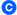Investment Appraisal (Cash Flow)
Net present value method, internal rate of return method etcAmortization
Monthly payment, principal and interest to date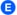General and Function
Virtually the same functions as a standard calculator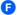Statistical and Regression
Statistical calculations using input sample dataInterest Rate Conversion
Nominal interest rate and effective interest rate conversion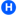Cost, Selling Price or Margin
Calculation of any of the above values after inputting the other two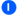Day or Date Calculations
Virtually the same as a standard calculatorDepreciation
Straight line-method, declining balance methodBond Calculation
Purchase price, annual yieldBreak-Even Point
Six modes for calculation of break-even point, etc

Qty